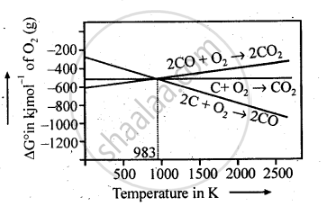PUC Karnataka Science Class 12Department of Pre-University Education, Karnataka
Share

Books Shortlist

Out of C and CO, Which is a Better Reducing Agent at 673 K? - PUC Karnataka Science Class 12 - Chemistry

ConceptExtraction of Crude Metal from Concentrated Ore

Question

Out of C and CO, which is a better reducing agent at 673 K?

Solution 1

At 673 K, the value of triangleG_(CO,CO_2) is less than that of triangleG_(("C,CO")) Therefore, CO can be oxidised more easily to CO2 than C to CO. Hence, CO is a better reducing ag ent than C at 673 K.

Solution 2

This can be explained thermodynamically, taking entropy and free energy changes into account.

C(s) + O_2(g) -> CO_2(g)

2C(s) + O_2(g) -> 2CO (g)

Case i: Volume of CO2 produced  = Volume of O2 used.

:.triangleS is very small and triangleG does not change with temperature.

:.Plot of triangleG Vs T is almost hrizontal

Case ii: Volume of CO produced = 2 x Volume of O2 used

:.triangleS is positive and hence triangleG beccomes increasingly negative as the temperature increases.

:.Plot of triangle ""^@G Vs T slopes downwards.As can be seen from ΔG° Vs T plot (Ellingham diagram), lines for the reactions, C ——–> C02 and C ——–> CO cross at 983 K. Below 983 K, the reaction (a) is energetically more favourable but above 673 K, reaction (b) is favourable and preferred. Thus, below 673 K both C and CO can act as a reducing agent but since CO can be more easily oxidised to C02 than C to C02 , therefore, below 673 K, CO is more effective reducing agent than carbon

Is there an error in this question or solution?

APPEARS IN

NCERT Solution for Chemistry Textbook for Class 12 (2015 to Current)
Chapter 6: General Principles and Processes of Isolation of Elements
Q: 5 | Page no. 163
Solution Out of C and CO, Which is a Better Reducing Agent at 673 K? Concept: Extraction of Crude Metal from Concentrated Ore.
S